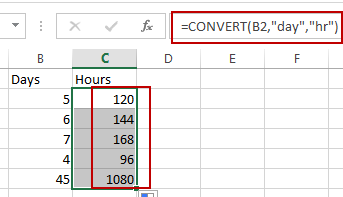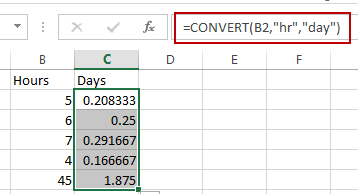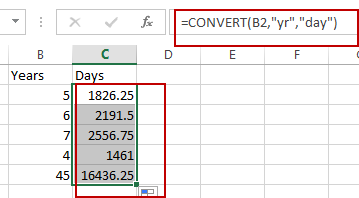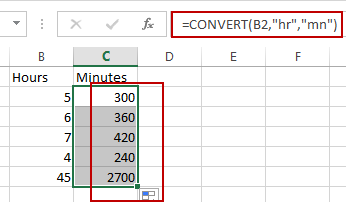# How to Convert Times in Excel

This post will guide you how to convert hours into minutes with a formula in Excel. How do I convert time to hours, minutes, or seconds or days in Excel.

## Convert between Time Units

If you want to find out how many hours there are in n days, or how many days are in n years in Excel, you can use a formula based on the CONVERT function to convert time between time units.

Convert Days to Hours

Assuming that you have a list of data in range B1:B5, in which contain day values, and if you want to convert all days to hours in a given range, you can use the CONVERT function to create the following formula to achieve it.

`=CONVERT(B2,"day","hr")`

Type this formula into a blank cell and press Enter key, and then drag the AutoFill Handle over to other cells to apply this formula.Convert Hours to Days

Assuming that you have a list of data in range B1:B5, in which contain Hour values, and if you want to convert all hours to days in a given range, you can use the CONVERT function to create the following formula to achieve it.

`=CONVERT(B2,"hr","day")`

Type this formula into a blank cell and press Enter key, and then drag the AutoFill Handle over to other cells to apply this formula.Convert Years to Days

If you want to convert Years to days, just use the following formula:

`=CONVERT(B2,"yr","day")`Convert Hours to Minutes

If you want to convert Hours to Minutes, just use the following formula:

`=CONVERT(B2,"hr","mn")`